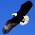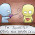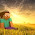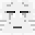## Monday, 25 March 2013

### Algebra: Who is right: Antonio, Benedict or Celine?

Antonio claims, " (a+b)^2 is always greater than a^2 + b^2 "
Benedict thinks, " Sometimes "
Celine says, " No! "

Now who is right?

You have been assigned the role of Antonio, Benedict and Celine.
You are going to tell people why you make the claims or think in such a manner:
• If you are Antonio, give examples to tell people that you are correct about your claim.
• Benedict sits on the fence. If you are Benedict, tell people why you think so?
• Celine claims that (a+b)^2 cannot be greater than a^2 + b^2. If you are Celine, then you have to tell people why you think in this manner.

Clue:
We can use a spreadsheet (i.e. NUMBERS) to help us.
Approach: we are going to substitute "a" and "b" with a range of numbers to "prove" our point.
Remember, one set of number is not enough to prove that what you say is always true. You will need to test it out with several sets of numbers.

• Set the headings for: a, b, a^2, b^2, a^2+b^2, a+b, 2ab
• Apart from a and b, use "formula" feature in the spreadsheet to help you compute the numbers.

1.[ I am Celine ] because if either a or b is a negative number then ( a + b )^2 would be smaller than a^2 + b^2 because ( a + b )^2 = a^2 + b^2 + 2ab and 2 x negative x positive is a negative therefore you would have to do subtraction from a^2 + b^2 thus making ( a + b )^2 smaller

2.I am Antonio :if a is zero and b is 1 ,both final answers would be 0 so i think the would be the same
but i still think benedict is right

3.( I am Benedict ) Look, if a or b is a negative number then (a+b)^2 is less. However, if say a and b is 0, then they would be equal.
And if both a and b are positive, then (a+b)^2 is more. Voila.

4.I am Celine. (a+b)^2 would be smaller than a^2 + b^2 if a or b is a negative number.

5.-I am Celine-
(a+b)^2 cannot be greater than a^2 + b^2 in situations where a or b is a -neg
For example,
a is -2 and b is -4 then...
(a+b)^2=(-2+(-4))^2
= 36
a^2 + b^2= -2^2 - 4^2
= -20

In conclusion,
-20 is smaller than a positive 36.

6.I am Celine

Assuming a is -1 and b is -2

(a+b)^2 = 1 + (-6) + 4
= -1

a^2 + b^2 = (-1)^2 + (-2)^2
= 1 + 4
= 5

In this case, (a+b)^2 is smaller than a^2 + b^2

Assuming a is 1 and b is -1

(a+b)^2 = 0

a^2 + b^2= 1 + 1
= 2

In this case, (a+b)^2 is also smaller than a^2 + b^2

7.( I am Benedict ) If a or b is a negative number then (a+b)^2 is less. If a and b is 0, then they would be equal.
If both a and b are positive, then (a+b)^2 will be more.

8.( I am Celine). If either of a or b is a negaive number, the statement will be wrong.

1.So (a+b)^2 cannot be greater than a^2 + b^2

9.I am Celine. (a+b)^2 can be smaller than a^2+b^2

Let a be 1 and let b be -1.

(a+b)^2 = (1+(-1))^2
= 0

a^2+b^2 = 1^2 + (-1)^2
= 1+1
= 2

In this case, a^2 + b^2 > (a+b)2

10.by ^2 it means to ^ the whole product. so what some think its 5x2+7x2=(5+7)x2, so some will apply that concept. on the other hand, let say (2+3)^2 is not 2^2+3^2

11.I am Celine.
Lets say either a or b would be a negative number,
then, (a+b)^2 would be smaller than (a+b)^2

12.I AM ANTONIO.
(a+b)^2 = a(a+b)+b(a+b) = a^2 +b^2 + 2ab.
a^2 +b^2 + 2ab is bigger than a^2+b^2

13.[I am Antonio](a+b)^2 is a^2 + b^2 +2ab.
a^2 + b^2 is smaller that (a+b)^2.
even if we assume a=1 and b=2,
(a+b)^2. is greater than a^2 + b^2.

14.BENEDICT
If a or b is a negative number, then a^2+b^2 will be more than (a+b)^2. But if a and b are positive than it would be vice versa.

15.I am benedict. It depends on the number If a and b are positive integer, then (a+b)^2 will be larger than a^2+b^2. But on the other hand, if a and b are negative integers or an integer(zero), then they will be the same. So yeah.

16.I am Benedict. Antonio is only correct when a and b are positive numbers. When a and b are 0, (a+b)^2 and a^2+b^2 have the same value. When a and b are negative numbers, a^2+b^2 is larger.

17.I am Antonio. (a+b)^2=a^2+2ab+b^2
a^2+2ab+b^2>a^2+b^2

18.( I am Benedict ). If one of the numbers a or b is 0, the equation is the same.But when the values, a and b has any value that is not 0, the equation will be the same.

19.I am Benedict. and it is true sometimes. For example, when (a) is 0 and (b) is 0 and when you square both of them a^2+2ab+b^2 you will get 0 which is the same a^2 +b^2

20.I am Antonio
if a is 1 and b is 2 , (1+2)^2 is greater than 1^2 + 1^2
but benedict is also right

21.This comment has been removed by the author.

22.I am Antonio. Here are some examples to show that it is greater.

(3+2)^2=25 3^2+2^2=13

(1+3)^2=16 1^2+3^2=10

23.I am Celine.
(a+b)^2
=(a+b)(a+b)
=a^2+b^2+2ab
If either a or b is a negative number, (a+b)^2 willbe smaller than a^2+b^2.